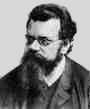Home

PHY 418: Statistical Mechanics I
Prof. S. Teitel stte@pas.rochester.edu ---- Spring 2018

## Problem Set 1

Due Monday, February 12, in lecture

• Problem 1 [35 points total]

Consider the classical ideal gas. In lecture, we found that the total entropy could be written as:

S(E,V,N) = (N/No)So + NkB ln [ (E/Eo)3/2(V/Vo)(N/No)-5/2 ]

where E is the total internal energy, V is the total volume, and N is the number of particles. Eo, Vo, No, So, and kB are constants.

(a) Starting from the above S(E,V,N), find the Helmholtz free energy A(T,V,N), the Gibbs free energy G(T,p,N), and the Grand Potential Σ(T,V,µ), by the method of Legendre transforms. [10 points]

(b) Find the familiar equation of state, pV = NkBT, by taking an appropriate 1st derivative of an appropriate thermodynamic potential. [5 points]

(c) Find the chemical potential µ, by taking an appropriate first derivative of a thermodynamic potential. By comparing to your result in part (a), show explicitly that the chemical potential is the same as the Gibbs free energy per particle. [5 points]

(d) Find the pressure p, by taking an appropriate first derivative of a thermodynamic potential. By comparing to your result in part (a), show explicitly that the pressure is the same as the negative of the Grand Potential per volume. [5 points]

(e) By computing the appropriate 2nd derivatives of the appropriate thermodynamic potentials, compute the specific heats CV and Cp, the compressibilities κT and κS, and the coefficient of thermal expansion α. Show by direct comparison of these results that the two specific heats, and the two compressibilities, are indeed related to each other by the general formulae derived in lecture. [5 points]

(f) If one allows the gas to expand isothermally (i.e. at constant temperature), how does the volume vary with the pressure? If one allows the gas to expand adiabatically (i.e. at constant entropy), how does the volume now vary with the pressure? You must show how you derive your results. [5 points]

• Problem 2 [10 points total]

Consider taking the Legendre transform of the energy, E(S,V,N), with respect to S, V, and N, to get a new thermodynamic potential, X(T,p,µ).

(a) Show that X(T,p,µ) is identically zero. [5 points]

(b) What does this imply about the variables T, p, and µ? Where have you already seen this result before? [5 points]

• Problem 3 [10 points total]

Prove the following relationship between the specific heat at constant pressure, Cp, and the coefficient of thermal expansion α.

(∂Cp/∂p)T = −TV[α2 + (∂α/∂T)p]
• Problem 4 [15 points total]

In a particular engine a gas is compressed in the initial stroke of the piston. Measurements of the instantaneous temperature, carried out during the compression, reveal that the temperature increases according to the relation:

T = (V/Vo)ηTo

where To and Vo are the initial temperature and volume and η is a constant. The gas is compressed to the volume V1 (where V1 < Vo). Assume that the gas is a monatomic ideal gas of N atoms, and assume the process is quasi-static (i.e. the system is always instantaneously in equilibrium).

a) Calculate the mechanical work done on the gas. [5 points]

b) Calculate the change in the total energy of the gas. [5 points]

c) Calculate the heat transfer Q to the gas. For what value of η is Q = 0? Show that this corresponds to the case of adiabatic compression (see Problem 1f). [5 points]

[Hint: you may use the facts you know about an ideal gas, i.e. pV = NkBT, and E = (3/2)NkBT.]